Show TOC

###Scrap Variance#### Use

The scrap variance is the value of the scrap variance quantity. The scrap variance quantity is the difference between the target scrap quantity (planned scrap quantity converted to yield) and actual scrap quantity (confirmed scrap quantity). The scrap variance quantity is valuated with the target cost from which the planned scrap costs have been deducted.

#### Integration

You can transfer the scrap variances to a value field in Profitability Analysis (CO-PA).

The scrap variances are part of the order balance that you can transfer to Financial Accounting (FI), Profit Center Accounting (EC-PCA), and Actual Costing/Material Ledger (CO-PC-ACT).

Note also the capabilities of user-defined error management .

#### Prerequisites

You must specify that the scrap variance is calculated by the variance calculation function. You do this by going into Customizing for Product Cost by Order or Product Cost by Period under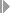Period-End ClosingVariancesDefine Variance Keys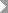and selecting the Scrap indicator.

You must specify that scrap variances are shown in variance calculation and that the control costs are calculated by deducting the scrap variances from the actual costs. You do this by going into Customizing for Product Cost by Order or Product Cost by Period underPeriod-End ClosingVariancesCheck Variance Variantsand selecting the Scrap indicator.

You can specify a valuation variant that controls the valuation of scrap in Customizing for Product Cost By Order or Product Cost By Period underPeriod-End ClosingVariancesDefine Valuation Variant for Work in Process and Scrap (Target Costs). You specify the valuation variant for scrap in target cost version 0. If you don’t define a valuation variant for scrap, the scrap variance is valuated at standard cost. The valuation variant for work in process and scrap is also used to valuate the work in process at target cost in Product Cost by Period .

In the Product Cost by Period component, SAP recommends valuating the scrap on the basis of the preliminary cost estimate for the product cost collector , particularly in repetitive manufacturing.

In repetitive manufacturing environments, make sure that the reporting point structure that you are using in production matches the reporting point structure you are using to calculate the target costs. If you change the reporting point structure, refer to the sections Flexible Reporting Points and Updating the Preliminary Cost Estimate .

Make sure that the cost estimate used to valuate the scrap is based on the same task list type and task list group as:

• The routing specified in repetitive manufacturing in the production version

• If manufacturing orders are being used, the routing in the manufacturing order

Every cost estimate that is relevant to the valuation of scrap variances must be a product cost estimate and have an itemization .Note

If you valuate scrap variances on the basis of a preliminary cost estimate for the manufacturing order, the itemization is generated dynamically when the variances are calculated.

This may have adverse effects on performance.

Valuating scrap variances on the basis of a preliminary cost estimate for the manufacturing order is not possible if you are collecting the costs of the manufacturing order on the product cost collector rather than on the manufacturing order itself.

End of the note.

#### Features

Scrap variances are always calculated in accordance with the valuation variant for work in process and scrap specified in target cost version 0. Scrap variances can be used to calculate the net actual costs with all target cost versions that have the reference base "actual." Check the settings in Customizing in the variance key and in the variance variant for this purpose.

The scrap variance are calculated by valuating the difference between the target scrap quantities and the scrap quantities confirmed at the operations with the target costs from which the costs for planned scrap have been deducted. The target scrap quantities are determined on the basis of the cost estimate used to calculate target costs, such as the standard cost estimate for the material or the preliminary cost estimate for a production order. If you have not planned any operation scrap in the routing, the value of the confirmed scrap quantity is reported as a scrap variance.

The valuation variant for work in process and scrap enables you to control which cost estimate is used to valuate the scrap variance:

• Planned costs/preliminary cost estimate (preliminary cost estimate for manufacturing order or product cost collector)

• An alternative material cost estimate (such as a modified standard cost estimate for calculation of the planned price of the period)

• A current standard cost estimate :

• In make-to-stock production, this is the standard cost estimate for the material.

• In sales-order-related production with a valuated sales order stock, this is the cost estimate used to calculate the standard price.

If you haven’t defined a valuation variant for the valuation of scrap variances, the system valuates the scrap variances automatically using the cost estimate that was used to calculate the standard price. In make-to-stock production, this is the standard cost estimate for the material. If you are using a valuated sales order stock, the standard price is determined using a predefined strategy (see also: Standard Price with Valuated Sales Order Stock ). The system valuates the scrap variance with the target cost calculated on the basis of the standard cost of the operation and from which the cost of planned scrap was deducted.

The cost estimate used as a basis for the valuation of the scrap variance may contain costs such as sales and administration costs that are not relevant for inventory valuation. In cost estimates with quantity structure, these costs are shown in a separate cost component view . Costs that are not relevant for inventory valuation are not included in the valuation of the scrap variance.

If you are using production orders, you can:

• Confirm scrap when you confirm the production order (that is, the order header)

When scrap is confirmed for the production order, the scrap quantity for each order is valuated with the net target cost per unit of measure.

• Confirm scrap when you confirm the operations of the production order

In repetitive manufacturing environments, you can confirm scrap quantities with reporting point backflushes.

When you confirm scrap for operations, the scrap variance quantity for each operation is valuated with the target cost of the operation from which the planned scrap was deducted.

When you confirm scrap for reporting points, the scrap variance quantity for each reporting point is valuated with the target cost of the operation from which the planned scrap was deducted.

#### Calculation of Target Costs on Basis of Standard Cost Estimate for Material

If you confirm on the basis of a standard cost estimate for the material and valuate work in process and scrap correspondingly, there are a number of different cases:

Case 1:

The current routing for the production version and the standard cost estimate for the material:

• Access the same BOM

• Have the same task list type

• Have the same routing group

• Have the same group counter

The work in process and scrap can be correctly valuated.

Case 2:

The current routing for the production version and the standard cost estimate for the material:

• Access the same BOM

• Have the same task list type

• Have the same routing group

• Have different group counters, but the reporting point structure is similar

You can use user-defined error management to ensure that work in process and scrap variances are calculated. The valuation of work in process and scrap is basically correct.

Case 3:

The current routing for the production version and the standard cost estimate for the material:

• Access the same BOM

• Have the same task list type

• Have the same routing group

• Have different group counters, and the reporting point structure is completely different

You can use user-defined error management to ensure that work in process and scrap variances are calculated. You can valuate the work in process and scrap, but the result will not be correct.

Case 4:

The current routing for the production version and the standard cost estimate for the material:

• Access the same BOM

• Have different task list types, or

• Have different routing groups

In this case it is not possible to calculate work in process or scrap at all.Note

To avoid the problems of cases 3 and 4, SAP recommends confirming and valuating work in process and scrap on the basis of the preliminary cost estimate.

End of the note.

Constraints

• You can specify a scrap quantity when you enter the final backflush. This quantity is currently not included when the scrap variance is calculated.

• You cannot calculate scrap variances for product cost collectors in sales-order-related production .

• You cannot calculate scrap variances for production orders created in CO ( production orders without quantity structure ).

• If a routing of a manufacturing order specifies a sequence of operations defined as a parallel sequence, the system cannot valuate the scrap variance. In this case no scrap variance will be reported when the variances are calculated. Instead, the costs for scrap variances are classified as remaining variances. If you are using reporting points in repetitive manufacturing, parallel sequences are not possible. This constraint is therefore irrelevant in repetitive manufacturing.

• If the Origin indicator in the material master record is selected, all material components must have homogeneous units of measure. For example, if you assign two raw materials with different units of measure (such as liters and pieces) to a cost element, meaningful summarization is not possible.

• If you have manually maintained assembly scrap but have not planned any operation scrap , the last operation of the routing is processed in Production Planning (PP) as if operation scrap had been maintained that caused the assembly scrap.

If a cost estimate is created on the basis of the routing, the quantities are increased in the cost estimate in accordance with this "simulated" operation scrap. The quantities of the planned (simulated) operation scrap are updated in the itemization.

These quantities also are included when the scrap variance is calculated. This means that the unplanned scrap quantity in such a case is the difference between the actual scrap quantity and the simulated planned operation scrap. The scrap variance quantity is valuated with the target costs less the simulated planned scrap quantities.

Example 1

Example for Scrap Variance When No Operation Scrap Was Planned

The following example valuates the scrap variance using the standard cost estimate for the material.

The standard cost estimate calculates the standard cost of the material being manufactured for each operation. The routing determines which operation the material components in the BOM are staged at (that is, which operation the goods issues and therefore the costs for the material components are assigned to).

Operations 0010 and 0030 of a production order are defined as milestones. No operation scrap was planned.

 Oper . Input qty / tons Target cost of operation Confirmed yield in tons Confirmed actual scrap qty in tons Scrap variance qty in tons Value of scrap variance in EUR 10* 2 l 15 min EUR 150 9 1 1 1 * 150 = 150 0.25 * 150 = 37.5 20 5 l 10 min EUR 100 0.25 * 100 = 25 30* 15 min EUR 50 3 0.25 0.25 0.25 * 50 = 12.50 Total 225

*Milestones

In operation 0010, 2 liters of raw materials and 15 minutes of internal activities are used for each finished ton according to the preliminary cost estimate for the product cost collector. The cost of goods manufactured per ton for this operation is EUR 150.

In operation 0020, 5 liters of raw materials and 10 minutes of internal activities are used for each finished ton according to the preliminary cost estimate for the product cost collector. The cost of goods manufactured per ton for this operation is EUR 100.

In operation 0030, 15 minutes of internal activities are used for each finished ton according to the preliminary cost estimate for the product cost collector. The cost of goods manufactured per ton for this operation is EUR 50.

At the first milestone (operation 0010), 9 tons yield and 1 ton scrap are confirmed. At the second milestone (after operation 0030), 3 tons yield and 0.25 ton scrap are confirmed.

When the system calculates the variances, it valuates the unplanned scrap quantity with the target cost calculated on the basis of the standard cost in the standard cost estimate. The target costs are calculated for all executed operations and reduced by the value of the planned scrap (in our example, this value is zero). When it calculates the variances, the system updates this information to the production order for each cost element or for each cost element and origin group. Because no operation scrap was planned, the confirmed scrap quantity is the same as the unplanned scrap quantity.

The target cost for the unplanned scrap in operation 0010 is EUR 150 (1 ton x EUR 150). The target cost for the unplanned scrap in operation 0030 is EUR 37.50 (0.25 ton x EUR 150) + EUR 25 (0.25 ton x EUR 100) + EUR 12.5 (0.25 ton x EUR 50).

The value of the scrap variance is EUR 225 (150 + 75).

Example 2

Example for Scrap Variance When Operation Scrap Was Planned

Planned Scrap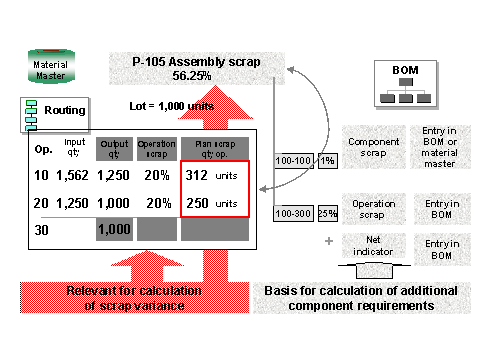( )

As in example 1, this example valuates the scrap variance using the standard cost estimate for the material.

In the routing, 20% operation scrap was planned for each of the two operations 10 and 20. This means that:

• It is anticipated that 20% of the units produced at operation 10 will be scrap.

• It is anticipated that 20% of the units produced at operation 20 will be scrap.

The planned operation scrap is reported in the itemization of the product cost estimate. This scrap is included in the determination of scrap variances.

Assembly scrap determined on the basis of the operation scrap increases the lot size of the material being produced. In this example, the assembly scrap is 56.25%.

Suppose that 100 kilograms of finished product are to be produced. To ensure that the final output is 100 kilograms, the system increases the lot size to 156.25 to account for the assembly scrap.

Assuming that the ratio of raw material used at operation 10 to finished product is 1:1, 156.25 kilograms of raw material enter the production process at operation 10. The output of operation 10 is 156.25 less 20% for the operation scrap or 31.25 kilograms, leaving 125 kilograms. Operation 20 incurs 20% scrap, or 25 kilograms. 100 kilograms enter the final operation (operation 30). Because no scrap was planned at operation 30, the output quantity also is 100 kilograms.

For detailed information on operation scrap in the routing, assembly scrap, component scrap, the net indicator, and on operation scrap in the BOM, as well as on the interactions between these factors, refer to the following section: Addendum: Scrap in Product Cost Controlling

Actual

During production, 312 kilograms of scrap are confirmed at operation 10 (as planned) and 300 kilograms of scrap are confirmed at operation 20.

Calculation of the Scrap Variance Quantity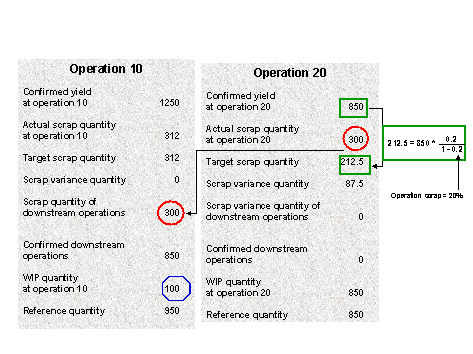( )

To determine the scrap variance, the system must first determine the scrap variance quantity (the quantity of unplanned scrap). The scrap variance quantity is determined by subtracting the actual scrap quantity from the target scrap quantity. The actual scrap quantity is the scrap quantity actually confirmed at an operation. The target scrap quantity is the planned scrap quantity that has been converted to the confirmed yield.

This example will examine operation 20 more closely.

The target scrap quantity is 212.5 kilograms. This amount is calculated as follows:

850 (confirmed yield) x 0.2 / (1 - 0.2) = 212.5 kilograms.

Result:

Actual scrap quantity 300 kilograms

- Target scrap quantity 212.50 kilograms

= Scrap variance quantity 87.50 kilograms (= quantity of unplanned scrap)

Calculation of the Scrap Variance (Costs)

The system determines the scrap variance by valuating the scrap variance quantity with the target cost less the planned operation scrap.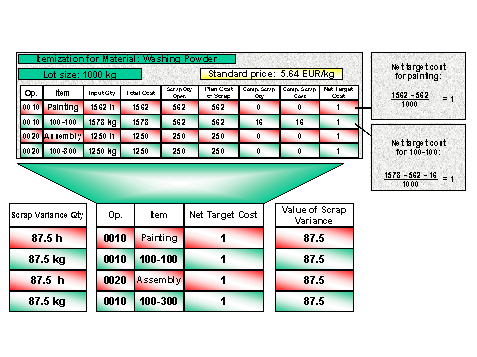( )

The cost of the planned scrap is deducted from the target cost with the following formula:

Net target cost =Total cost - Cost of planned scrap

Lot size

The net target costs are calculated for each costing item.

Explanation Facility for Scrap Variance

The explanation facility shows the reference quantity used to calculate the work in process (only with the calculation of work in process at target costs in the Product Cost by Period component) (see also the figure Calculation of Scrap Variance Quantity ). In the explanation facility, you can use the F1 help to assist you in interpreting the key figures.

For information on the WIP quantity and the reference quantity, refer to following section: Work in Process in Product Cost by Period

For information on how to access the explanation facility for scrap variances, refer to the following section: Calculating Variances